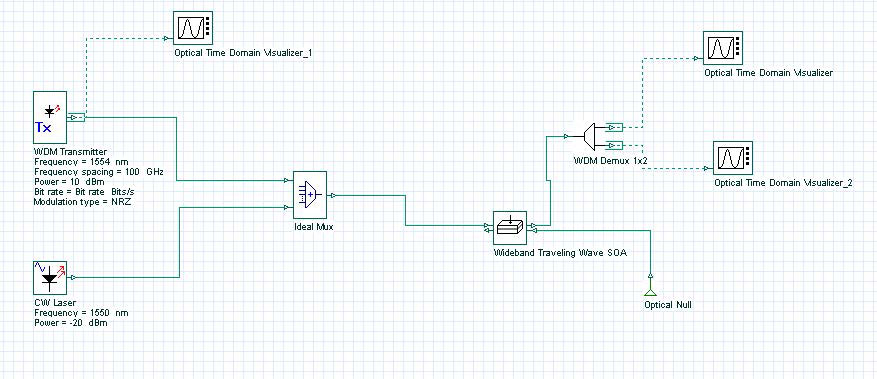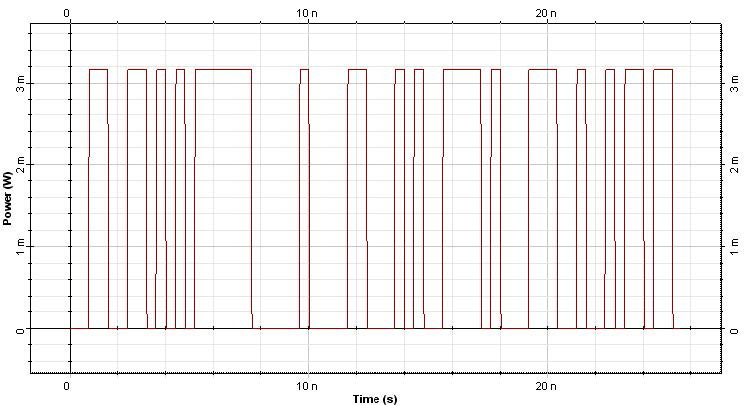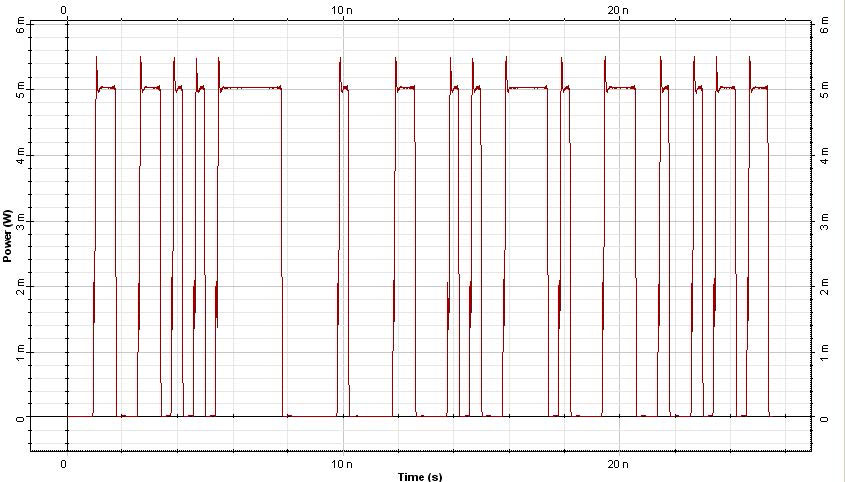The objective of this lesson is to demonstrate the wavelength conversion using the wideband SOA component.

Based on the results from the lesson “Wideband SOA Characterization”, we have designed a wavelength converter using the cross-gain modulation method.

The system designed is shown in Figure 1.Figure 1: Wavelength converter system layout

In the simulation, the original information modulated at the signal at 1554 nm will be converted to the wavelength at 1550 nm. See Figure 2.Figure 2: Original modulated signal at 1554 nm

Based on the cross gain modulation, the initial CW signal at 1550 nm is modulated at the SOA and the output signal at 1550 becomes the signal shown in Figure 3.Figure 3: Wavelength converted signal at 1550 nm